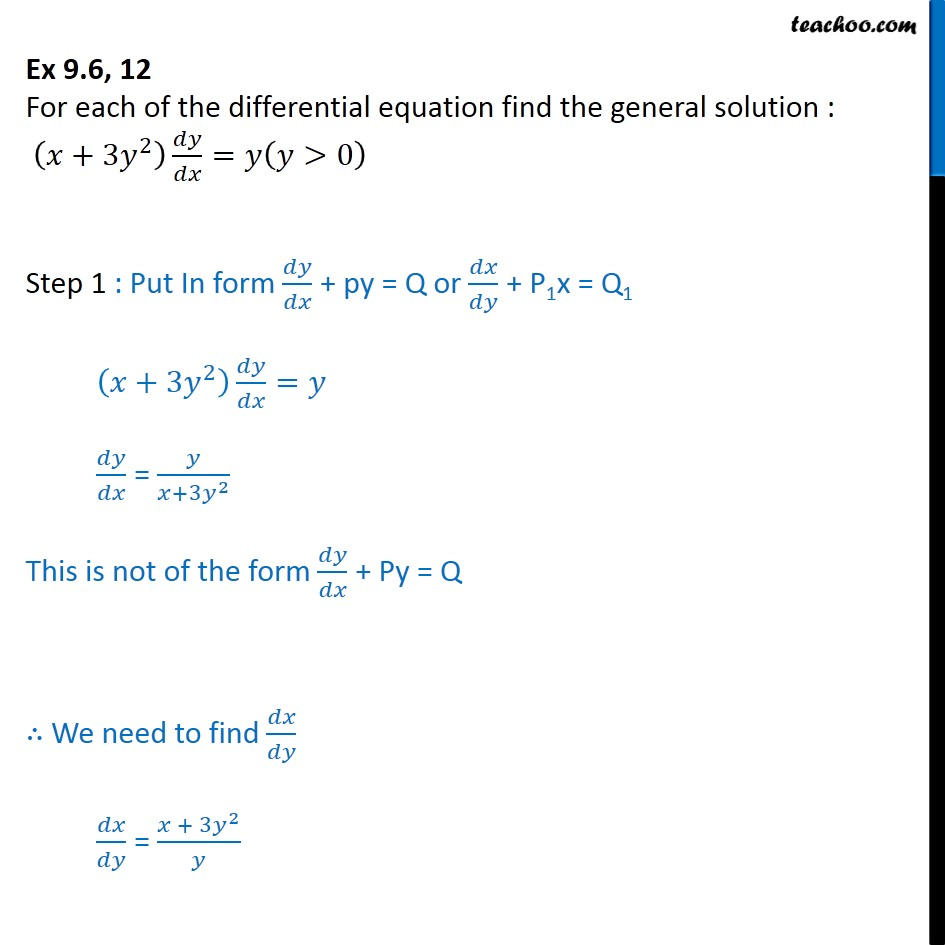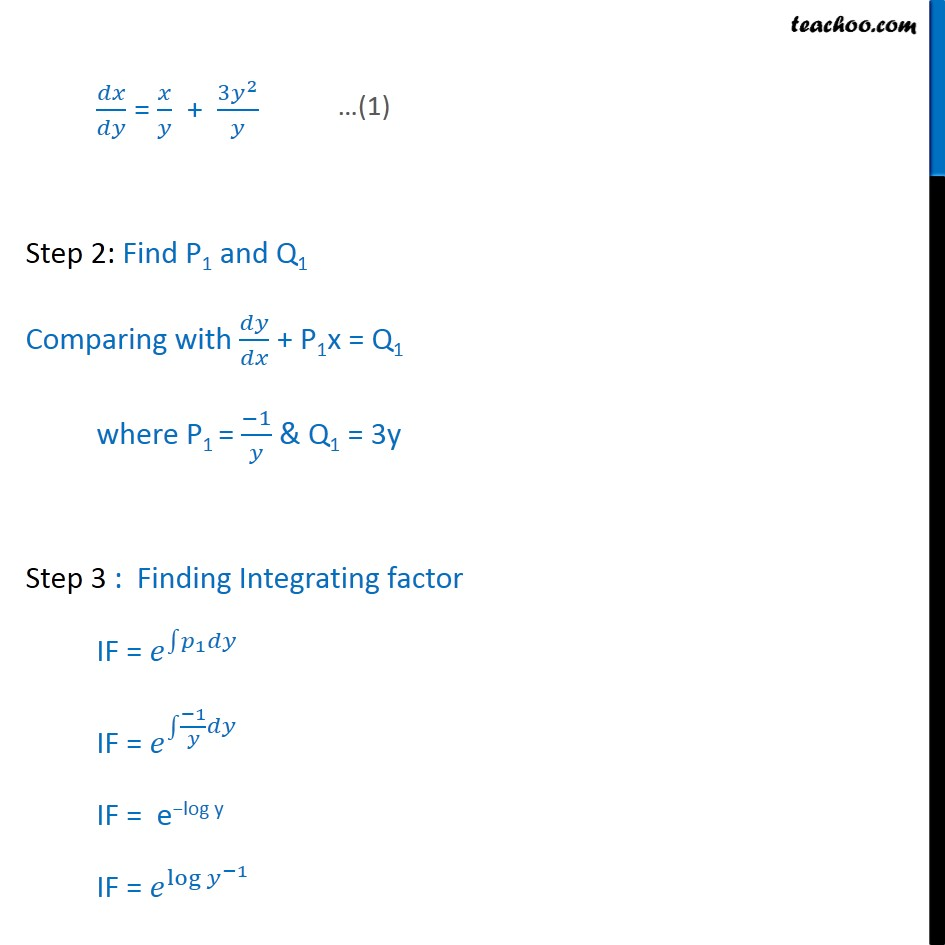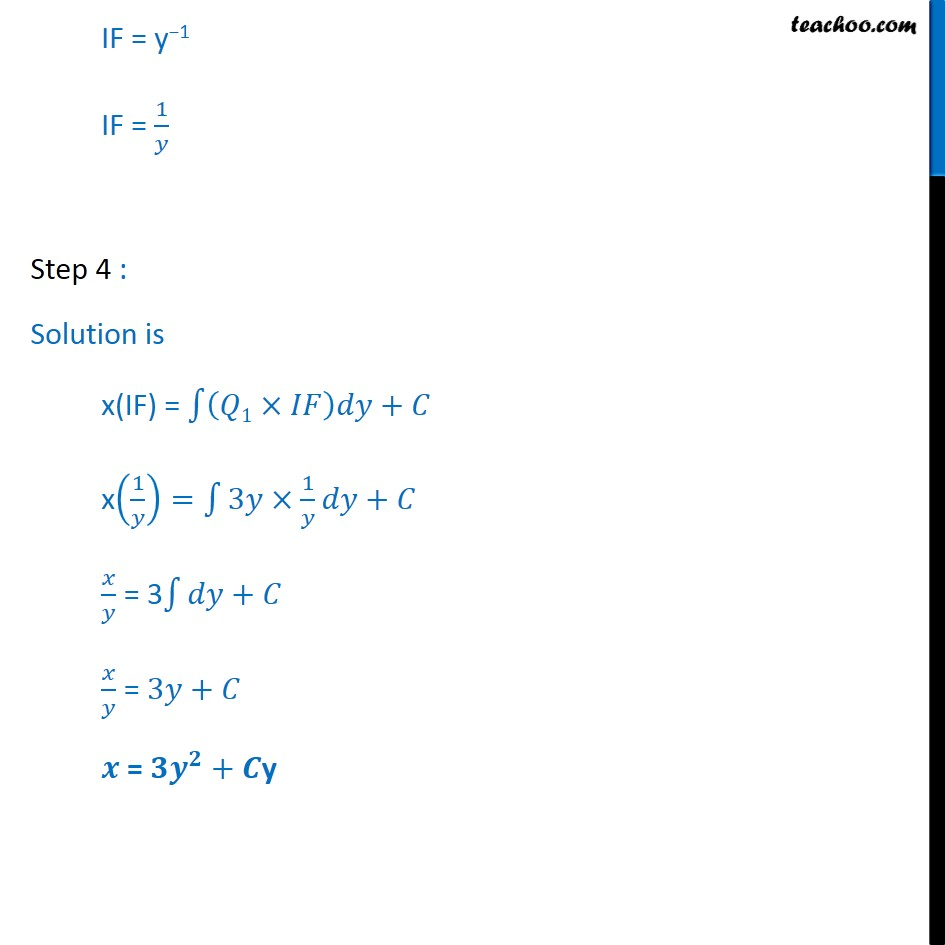Ex 9.5

Chapter 9 Class 12 Differential Equations
Serial order wiseLearn in your speed, with individual attention - Teachoo Maths 1-on-1 Class

### Transcript

Ex 9.5, 12 For each of the differential equation find the general solution : +3 2 = >0 Step 1 : Put In form + py = Q or + P1x = Q1 +3 2 = = +3 2 This is not of the form + Py = Q We need to find = + 3 2 = + 3 2 Step 2: Find P1 and Q1 Comparing with + P1x = Q1 where P1 = 1 & Q1 = 3y Step 3 : Finding Integrating factor IF = 1 IF = 1 IF = e log y IF = log 1 IF = y 1 IF = 1 Step 4 : Solution is x(IF) = 1 + x 1 = 3 1 + = 3 + = 3 + = + y